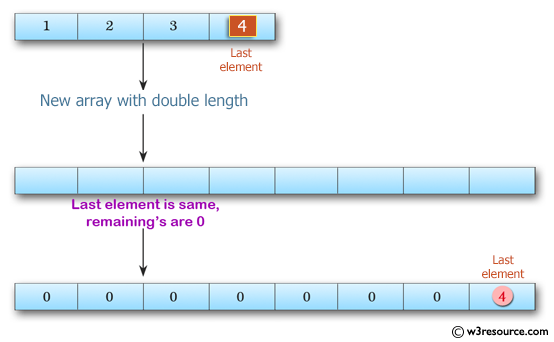﻿ Swift Array Programming Exercise: Create a new array with double the lenght of a given array of integers and its last element is the same as the given array - w3resource# Swift Array Programming Exercises: Create a new array with double the lenght of a given array of integers and its last element is the same as the given array

## Swift Array Programming: Exercise-13 with Solution

Write a Swift program to create a new array with double the lenght of a given array of integers and its last element is the same as the given array. The given array will be length 1 or more. By default, a new integer array contains all 0's.

Pictorial Presentation:Sample Solution:

Swift Code:

``````func new_array(_ a: [Int]) -> [Int] {
var new_array: [Int] = [a.last!]

for _ in a {
new_array.insert(0, at: new_array.startIndex)
new_array.insert(0, at: new_array.startIndex)
}
new_array.remove(at: 0)

return new_array
}

print(new_array([1, 2, 3, 4]))
print(new_array([1, 2, 3]))
print(new_array())
```
```

Sample Output:

```[0, 0, 0, 0, 0, 0, 0, 4]
[0, 0, 0, 0, 0, 3]
[0, 1]
```

Swift Programming Code Editor:

Improve this sample solution and post your code through Disqus

What is the difficulty level of this exercise?

﻿